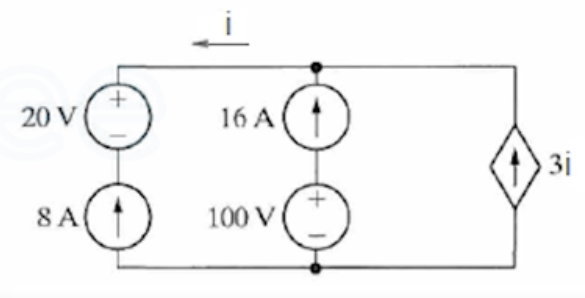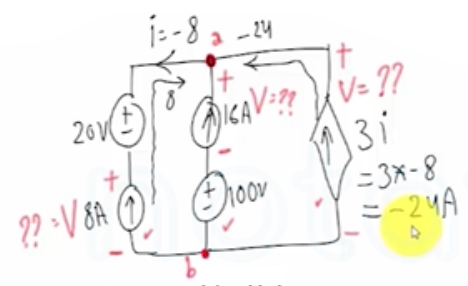Need Help?

Subscribe to Circuit

###### \${selected_topic_name}
• Notes

$\begin{array}{l}{\text { Using the definitions of the ideal independent and dependent sources, state which interconnections are }} \\ {\text { valid and which violate the constraints imposed by the ideal sources. }}\end{array}$

$\begin{array}{l}{\text { a) Is the interconnection in the shown Figure Valid? Explain. }} \\ {\text { b) Can you find the total energy developed in the circuit? Explain. }}\end{array}$$K CL$

$\Sigma I=0$

$i=-8$$\Sigma I=16-24-(-8)=0$

$E=P t=V I t$

$\text { Eneregy cant be calculated. }$